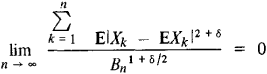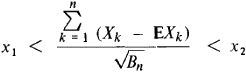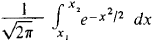# Liapunov Theorem

## Liapunov Theorem

in probability theory a theorem that asserts certain extremely general sufficiency conditions for the convergence of the distribution of sums of independent random variables to a normal law. It was formulated and proved by A. M. Liapunov in 1901. The Liapunov theorem completed the investigations of P. L. Chebyshev, A. A. Markov (the elder), and Liapunov himself along what proved to be a fundamental line of research for probability theory as a whole.

A precise formulation of the Liapunov theorem is as follows. Let the independent random variables X1,. .., Xn, … have finite mathematical expectations EXk, dispersions DXk, and, for δ > 0, absolute moments E|Xk-EXk|2+δ and let Bn = Σnk=1 be the dispersion of the sum X1,… ,Xn. We assert that if for some δ > 0(the Liapunov condition), then the probability of the inequalityapproaches, as n→∞, the limituniformly for all values of x1 and x2. Liapunov also provided an estimate of the rate of convergence in the Liapunov theorem. Subsequently, conditions were established that expanded the Liapunov condition and are not only sufficient but, in some sense, necessary.

### REFERENCES

Liapunov, A. M. “Novaia forma teoremy o predele veroiatnosti.” Sobr. soch., vol. 1, p. 157. Moscow, 1954.
Bernshtein, S. N. Teoriia veroiatnostei, 4th ed., Moscow-Leningrad, 1946. Page 275.

A. V. PROKHOROV

Site: Follow: Share:
Open / Close